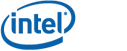# Voronoi Builder

The Voronoi builder is the event generator structure, that implements the sweepline algorithm, scanning 2D space and spawning the two types of events: site events and circle events. Each event is reported to the output data structure builder. The structure shares Voronoi name, as the events generated by it provide enough information to construct the Voronoi diagram of a set of points and linear segments. The requirements for the coordinate type of the input/output geometries, configured through the coordinate type traits template argument, are the following:
• The input coordinate type (for input points and enpoints of the input segments) is not required to be integral (while it still should be an integer type)
• The output coordinate type (for Voronoi vertices) is required to be IEEE-754 floating point type

## Important

The users are encouraged to use the default static methods defined in the voronoi.hpp header for the Voronoi diagram construction. However it's also possible to use Voronoi builder explicitly, especially if the user wants to drop the external dependencies such as MPL (metaprogramming library). The following include set doesn't depend on any external headers (except STL and boost/cstdint.hpp), even the Boost.Polygon library:

#include <voronoi_builder.hpp>
#include <voronoi_diagram.hpp>

## Declaration

template <typename T,
typename CTT = detail::voronoi_ctype_traits<T>,
typename VP = detail::voronoi_predicates<CTT> >
class voronoi_builder;

T
- specifies the coordinate type of the input geometries (points and segments).
CTT - defines the input/output coordinate type traits used by the Voronoi predicates (VP).
VP - the predicate kernel, that contains robust and efficient predicates and functors.
The Voronoi builder data structure is ready to use from the box with 32-bit signed integer input coordinate type. The user may extend the input coordinate range to the other integer types (e.g. 64-bit integer), however this will also require manual setup of the coordinate type traits. Default predicate kernel provides correct and efficient predicates as long as types defined by the coordinate type traits satisfy the requirements explained at the end of this page. In case those requirements are not satisfied, the proper predicate kernel implementation is required.

## Member Functions

 voronoi_builder() Default constructor. size_t insert_point(const int_type& x,                     const int_type& y) Inserts a point object with the specified coordinates into the Voronoi builder. Returns index of the inserted point. size_t insert_segment(const int_type& x1,                       const int_type& y1,                       const int_type& x2,                       const int_type& y2) Inserts a segment object with the specified coordinates into the Voronoi builder. Returns index of the inserted segment. template void construct(OUTPUT* output) Runs the sweepline algorithm over the set of inserted geometries and generates site and circle events to the OUTPUT data structure. It's the responsibility of the output data structure to process them. Complexity: O(N * log N), where N is the total number of input points and segments. void clear() Clears the list of the inserted geometries. Sets the input geometry index to zero.

# Voronoi Coordinate Type Traits

The library provides the default coordinate type traits specialization for the 32-bit signed integer type:

template <typename T>
struct voronoi_ctype_traits;

template <>
struct voronoi_ctype_traits<int32> {
typedef int32 int_type;
typedef int64 int_x2_type;
typedef uint64 uint_x2_type;
typedef extended_int<128> big_int_type;
typedef fpt64 fpt_type;
typedef extended_exponent_fpt<fpt_type> efpt_type;
typedef ulp_comparison<fpt_type> ulp_cmp_type;
typedef type_converter_fpt to_fpt_converter_type;
typedef type_converter_efpt to_efpt_converter_type;
};

One of the most important features of the library is that Voronoi builder output geometries are constructed within defined relative error (64 machine epsilons). That means, the more mantissa bits the user provided fpt_type has, the better precision of the output geometries will be. In order for the user defined traits to be consistent with the default Voronoi builder predicate kernel user should define the following set of traits (the assumption is made that input geometries have X-bit signed integer coordinate type):

 int_type At least X-bit signed integer type. int_x2_type At least 2X-bit signed integer type. uint_x2_type At least 2X-bit unsigned integer type. big_int_type At least 8X-bit signed integer type if input dataset contains only points. At least 64X-bit signed integer type if input dataset contains segments. fpt_type IEEE-754 floating point type, with mantissa at least (X+16) bits and exponent able to handle 32X-bit unsigned integer type. efpt_type IEEE-754 floating point type, with mantissa at least (X+16) bits and exponent able to handle 64X-bit unsigned integer type. ulp_cmp_type Ulp comparison structure, that checks if two fpt_type values are within the given ulp range (relative error range). to_fpt_converter_type Type converter structure, that converts any of the integer types above plus efpt_type to the fpt_type using operator(). to_efpt_converter_type Type converter structure, that converts any of the integer types above to the efpt_type using operator().

Notes:

• Four different integer types are used (instead of a single big_int_type) to slightly improve algorithm performance and memory usage.
• As the maximum required size of the big_int_type is known in advance, it's possible to use fixed, stack allocated, multiprecision integer type.
• Two separate floating-point types are defined, because for the input geometries with 32-bit signed integer coordinates, double type won't be able to handle 2048-bit (64 chunks of 32 bits each) integers, as they will overflow its exponent. On the gcc compiler it's possible to use 80-bit long doubles for both fpt types, however this is not supported by the MSVC compiler.
• efpt_type and to_efpt_converter_type are not used to construct the Voronoi diagram of a set of points (mock implementation will work).
• For an example of the user defined coordinate type traits check the advanced Voronoi tutorial.